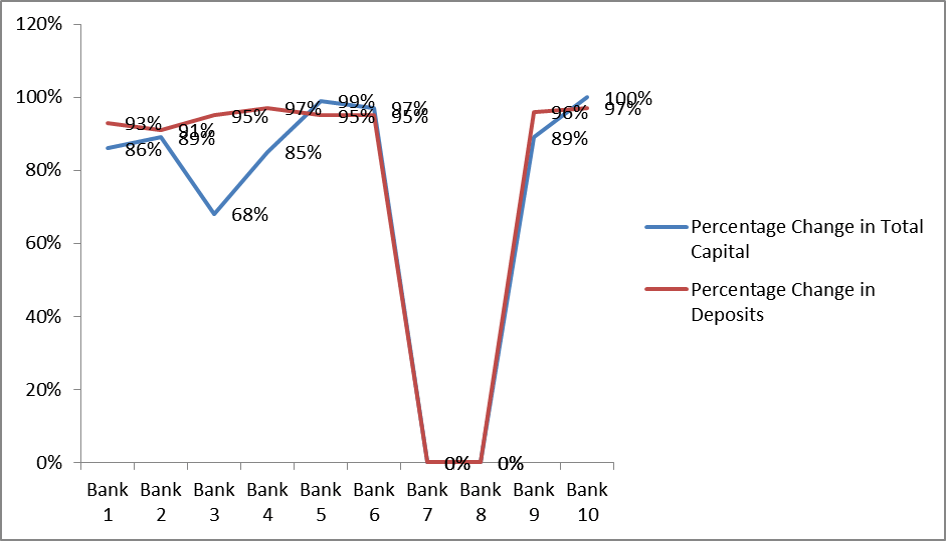# Multi-stage CRS analysis and interpretation of input orientation

By Avishek Majumder and Shruti Datt on September 5, 2017

In the previous article “Importing the data and defining the variable” the steps for data extraction and execution of results were discussed. Here, the findings for Multi-stage based constant returns to scale (CRS) are extracted and analyzed and presented. The findings and the interpretations are on the basis of input-oriented multi-staged CRS Data Envelopment Analysis. Input variables are Total Capital and Deposits while the output variables are Total loans and Profits.

## Comparative analysis of bank efficiency in CRS model

From the results of efficiency summary; banks 7 and 8. Thus, ICICI bank and HDFC bank has efficiency value of 1.000. Other banks have values less than 1.000 which indicates the need to improve their input variables. This is possible by either decreasing or increasing the input levels.

For example, in case of Allahabad bank, they need to improve its efficiency by 89.2% followed by SBI with 85.8%. In terms of other private banks, Axis banks need to improve its efficiency by 46%.

Thus, a bank is said to have efficient performance when the efficiency value is 1.

## Summary of Slacks

This is a value which shows the discrepancy in the constant or proportional change of input and output variables (Coelli, 2008). It also represents the amount of value for improvement in both input and output. The image below reflects that the 1st bank should either increase or decrease the deposits by 129597.661 to become efficient. Slacks only show the variable discrepancy between the constant output and input. It is only perceived that the value must be either increased or decreased (Cooper, Seiford, & Zhu, 2011). Similarly, the 6th bank can either increase or decrease their input of Total Capitals by 2102.055 to become efficient.

## Summary of Peers

The term Peer or Reference set indicates an inefficient firm to follow or as a reference (Coelli, 2008). The inefficient firms such as bank 1 can follow the input-output trend of any of 8 or 7. But the efficient banks don’t have to follow any firm. Furthermore, peer weight is a number of variables that can be followed for the respective reference firm (Cooper et al., 2011). For the 1st firm, since it has peers value for 7 and 8. Thus, it can follow 0.6% of the total capitals and deposits of bank 8. Furthermore, it can also follow 25% of deposits of the bank 7 to become efficient. Finally, peer count shows the number of times a peer firm is being used as the reference unit. The 8th bank has been used as a peer 5 times, while bank 7 is used 8 times.

## Summary of Targets

This shows the model that is to be followed for the preferred and perceived Total Capitals and Deposits that will help in making the case banks efficient (Cooper et al., 2011). However, the Total Capitals and Deposits (input targets) remain the same for efficient organizations. Thus, for Bank 1; for constant output of Total Loans and Profits, it must have an efficiently proportional Total capital of 710170.519 and Deposits of 98048.384. So Bank 1 can increase the financials of Total Capitals by 710170.519 and Deposits by 98048.384. Similar interpretations are possible for all inefficient banks.

Target value are different from slacks value. Slacks show discrepancies while Summary of targets show predicted value.

The graph below show the percentage of difference change. 0% change shows that the banks are efficient and do not need to change their values. For bank 1 it needs to change its total capital by 86% and deposits by 93% to become efficient. Similar interpretations are possible for other banks as well.Graph showing percentage change of Total Capital and Deposits as inputs for the banks to become efficient

## Radial Movement, Slack Movement, and Projected Value

The Radial Movement shows the adjusted proportionality of input and output variables (Coelli, 2008). Radial Movement gives the value for input-output variables for improvement (Coelli, 2008). The radial values for the bank 1 are -193903.733 for Total capital, Deposits is -1485544.680 and -129597.661. The projected value of input for the 1st bank against each output is 32002.267 (Total Capital) and 115579.658 (Deposits).

Bank 1 can adopt Total Loans of 1870260.000 and Total profit of 258214.000 output values to achieve efficiency. The values are to be decreased by -193903.733 and -1485544.680 for Total Capital and Deposits respectively. Similarly, interpretations are possible for other inefficient firms. Projected value shows the proportionality of input and output for every radial and slack movement.